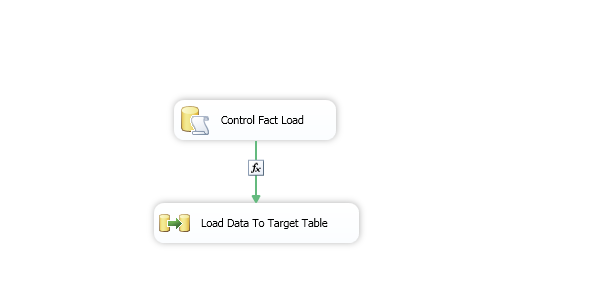## Friday, April 21, 2017

### SSIS Tricks: Control executing the next SSIS tasks

Your SSIS package may requrie to have control that will make decision if the next SSIS tasks will execute or not. I have an example where 'Data Flow Task' for loading the fact table will start if 'Execute SQL  Task' return TRUE.

Here as an example; I have a sequence container that look like below:Fig 1: Execute SQL task and  Data flow task
Let's look at the code in SQL Execute task named "Control Fact Load":Fig 2: SQL query for SQL Execute Task

The SQL code in the above diagram:

SELECT case when max([TimeKey])<convert(varchar(8),getdate(),112)
Then 'Y'
Else 'N'
END  as GoToNextStep
FROM [dbo].[Fact_XXX]

The SQL code in the above diagram intend to find if there is any Date exist in the fact table bigger than today. It means if the fact table's date "TimeKey" is less than today's date then we retutn 'Y' ; so, we did not load any data for today yet. And if today's date is already exist it means data is uploaded to the fact already then we return 'N' hence next SSIS task will not execute.

The return value of the SQL need to save in a variable. So user variable GoToNextStep is created and assigned the value after the Execute SQL task as like below:
And then assigned the value after SQL execute task:

Fig 5: Binding value to the variable

The way we control between two tasks is precedence constraint.Fig 6: Prcedence constraint editior

You need to open the prcedence constraint editior (right click the connector between two tasks) and then set the value as like below:
Evaluation operation: Expression and Constraint
Value=Success
Expression: @[User::GoToNextStep]=="Y"

Now, Data Flow Task (Load Data To Target table) will only execute if prior Exceute SQL task (Control Fact Load) return "Y". If 'N' is returned via Exceute SQL task (Control Fact Load) then next data flow task (Load Data To Target table) will not execute at all.